# Highlight the maximum value in each column in Pandas

Let’s discuss how to highlight the maximum values in Pandas Dataframe. Lets first make a dataframe:

Example:

## Python3

 `# Import Required Libraries ` `import` `pandas as pd ` `import` `numpy as np ` ` `  `# Create a dictionary for the dataframe ` `dict` `=` `{``'Name'``: [``'Sukritin'``, ``'Sumit Tyagi'``, ``'Akriti Goel'``, ` `                 ``'Sanskriti'``, ``'Abhishek Jain'``],  ` `        ``'Age'``: [``22``, ``20``, ``45``, ``21``, ``22``], ` `        ``'Marks'``: [``90``, ``84``, ``33``, ``87``, ``82``]} ` ` `  `# Converting Dictionary to Pandas Dataframe ` `df ``=` `pd.DataFrame(``dict``) ` ` `  `# Print Dataframe ` `df`

Output: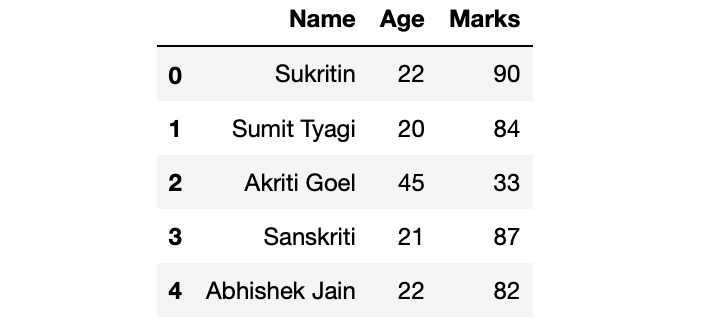Now, come to the highlighting part. Our objective is to highlight cells with maximum values in each column.

Method 1: Highlighting Cell with maximum value in each column

We will do this by using the highlight_max() method of DataFrame property. highlight_max() method takes 3 arguments, subset = name of the columns of which you want to find the maximum, color = name of the color with which you want to highlight the cell and axis = (0/1) based on which axis you want find the maximum.

## Python3

 `# Highlighting the maximum values of ` `# last 2 columns ` `df.style.highlight_max(color ``=` `'lightgreen'``, axis ``=` `0``)`

Output: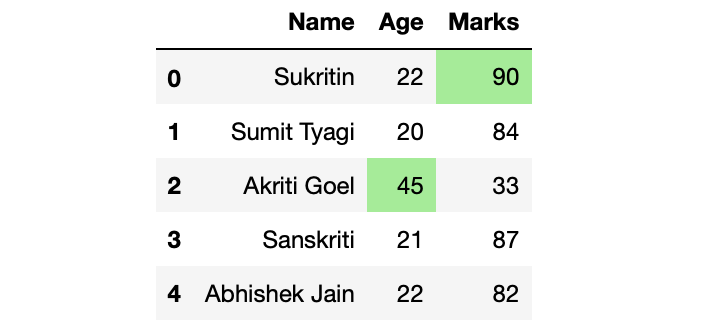Method 2: Highlighting the text instead of cell

## Python3

 `# Defining custom function which returns  ` `# the list for df.style.apply() method ` `def` `highlight_max(s): ` `    ``is_max ``=` `s ``=``=` `s.``max``() ` `    ``return` `[``'color: green'` `if` `cell ``else` `'' ``for` `cell ``in` `is_max] ` ` `  `df.style.``apply``(highlight_max)`

Output: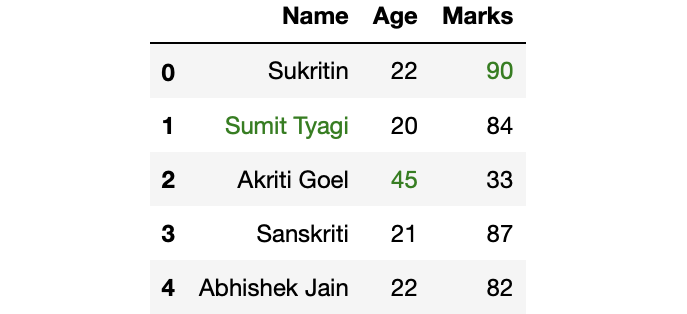Method 3: Highlighting cell with maximum values

## Python3

 `# Defining custom function which returns  ` `# the list for df.style.apply() method ` `def` `highlight_max(s): ` `    ``is_max ``=` `s ``=``=` `s.``max``() ` `    ``return` `[``'background: lightgreen'` `if` `cell ``else` `'' ``for` `cell ``in` `is_max] ` ` `  `df.style.``apply``(highlight_max)`

Output: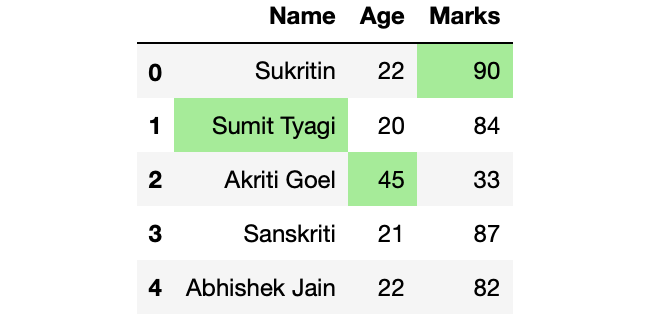Method 4: Highlighting cell with maximum values but not highlighting the string values

## Python3

 `# Defining custom function which returns ` `# the list for df.style.apply() method ` `def` `highlight_max(s): ` `    ``if` `s.dtype ``=``=` `np.``object``: ` `        ``is_max ``=` `[``False` `for` `_ ``in` `range``(s.shape[``0``])] ` `    ``else``: ` `        ``is_max ``=` `s ``=``=` `s.``max``() ` `    ``return` `[``'background: lightgreen'` `if` `cell ``else` `'' ``for` `cell ``in` `is_max] ` ` `  `df.style.``apply``(highlight_max)`

Output: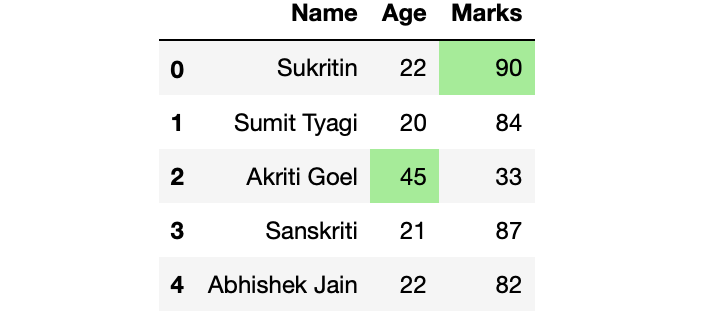My Personal Notes arrow_drop_upCheck out this Author's contributed articles.

If you like GeeksforGeeks and would like to contribute, you can also write an article using contribute.geeksforgeeks.org or mail your article to contribute@geeksforgeeks.org. See your article appearing on the GeeksforGeeks main page and help other Geeks.

Please Improve this article if you find anything incorrect by clicking on the "Improve Article" button below.

Article Tags :

1

Please write to us at contribute@geeksforgeeks.org to report any issue with the above content.